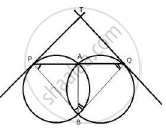Share

# Two Circles Intersect Each Other at Points a and B. a Straight Line Paq Cuts the Circles at P and Q. If the Tangents at P and Q Intersect at Point T; Show that the Points P, B, Q and T Are Concyclic - Mathematics

Course

#### Question

Two circles intersect each other at points A and B. A straight line PAQ cuts the circles at P and Q. If the tangents at P and Q intersect at point T; show that the points P, B, Q and T are concyclic

#### SolutionJoin AB, PB and BQ
TP is the tangent and PA is a chord
∴ ∠TPA = ∠ABP …… (i) (angles in alternate segment)
Similarly,
∠TQA = ∠ABQ ……. (ii)
∠TPA+ ∠TQA = ∠ABP + ∠ABQ
But, Δ PTQ,
∠TPA  + ∠TQA + ∠PTQ  =180°
⇒ ∠ PBQ  = 180° -  ∠PTQ
⇒  ∠PBQ  + ∠PTQ =  180° ...
But they are the opposite angles of the quadrilateral
Therefore, PBQT are cyclic.
Hence, P, B, Q and T are concyclic.

Is there an error in this question or solution?